Introduction to using R package: womblR

Use of womblR

This is a brief description of how to use the womblR package within the context of glaucoma progression. We begin by loading the package.

library(womblR)

In the womblR package there is a longitudinal series of visual fields that we will use to exemplify the statistical models contained in the package. The data object is called VFSeries and has four variables, Visit, DLS, Time and Location. The data object loads automatically; here’s what the data looks like,

##   Visit DLS Time Location
## 1     1  25    0        1
## 2     2  23  126        1
## 3     3  23  238        1
## 4     4  23  406        1
## 5     5  24  504        1
## 6     6  21  588        1

The variable Visit represents the visual field test visit number, DLS the observed outcome variable, differential light sensitvity, Time the time of the visual field test (in days from baseline visit) and Location the spatial location on the visual field that the observation occured. To help illuminate visual field data we can use the PlotVFTimeSeries function. PlotVFTimeSeries is a function that plots the observered visual field data over time at each location on the visual field.

PlotVfTimeSeries(Y = VFSeries$DLS, Location = VFSeries$Location,
Time = VFSeries$Time, main = "Visual field sensitivity time series \n at each location", xlab = "Days from baseline visit", ylab = "Differential light sensitivity (dB)", line.col = 1, line.type = 1, line.reg = FALSE)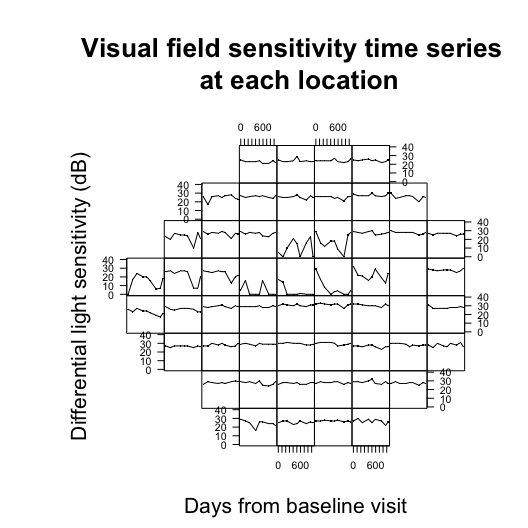The figure above demonstrates the visual field from a Humphrey Field Analyzer-II testing machine, which generates 54 spatial locations (only 52 informative locations, note the 2 blanks spots corresponding to the blind spot). At each visual field test a patient is assessed for vision loss. Format data for STBDwDM We can now begin to think about preparing objects for use in the the Spatiotemporal Boundary Detection with Dissimilarity Metric model function (STBDwDM). According to the manual, the observed data Y must be first ordered spatially and then temporally. Furthermore, we will remove all locations that correspond to the natural blind spot (which in the Humphrey Field Analyzer-II correspond to locations 26 and 35). blind_spot <- c(26, 35) # define blind spot VFSeries <- VFSeries[order(VFSeries$Location), ] # sort by location
VFSeries <- VFSeries[order(VFSeries$Visit), ] # sort by visit VFSeries <- VFSeries[!VFSeries$Location %in% blind_spot, ] # remove blind spot locations
Y <- VFSeries$DLS # define observed outcome data Now that we have assigned the observed outcomed Y we move onto the temporal variable Time. For visual field data we define this to be the time from the baseline visit. We obtain the unique days from the baseline visit and scale them to be on the year scale. Time <- unique(VFSeries$Time) / 365 # years since baseline visit
print(Time)
##  0.0000000 0.3452055 0.6520548 1.1123288 1.3808219 1.6109589 2.0712329
##  2.3780822 2.5698630

Our example patient has nine visual field visits and the last visit occured 2.57 years after the baseline visit.

We now specify the adjacency matrix, W, and dissimilarity metric, DM. There are three adjacency matrices for the Humphrey Field Analyzer-II visual field that are supplied by the womblR package, HFAII_Queen, HFAII_QueenHF, and HFAII_Rook. HFAII_Queen and HFAII_QueenHF both define adjacencies as edges and corners (i.e., the movements of a queen in chess), while HFAII_Rook only defines an adjacency as a neighbor that shares an edge (i.e., a rook in chess). The HFAII_QueenHF adjacency matrix does not allow neighbors to share information between the northern and southern hemispheres of the visual field. In this analysis we use the standard queen specification. The adjacency objects are preloaded and contain the blind spot, so we define our adjacency matrix as follows.

W <- HFAII_Queen[-blind_spot, -blind_spot] # visual field adjacency matrix

Now we turn our attention to assigning a dissimilarity metric. The dissimilarity metric we use in this data application are the Garway-Heath angles that describe the underlying location that the retinal nerve fibers enter the optic disc. These angles (measured in degrees) are included with womblR in the object GarwayHeath. We create the dissimilarity metric object DM.

DM <- GarwayHeath[-blind_spot] # Garway-Heath angles

The womblR package provides a plotting function PlotAdjacency that can be used to display a dissimilarity metric over the spatial structure of the visual field. We demonstrate it using the Garway-Heath angles.

PlotAdjacency(W = W, DM = DM, zlim = c(0, 180), Visit = NA,
main = "Garway-Heath dissimilarity metric\n across the visual field")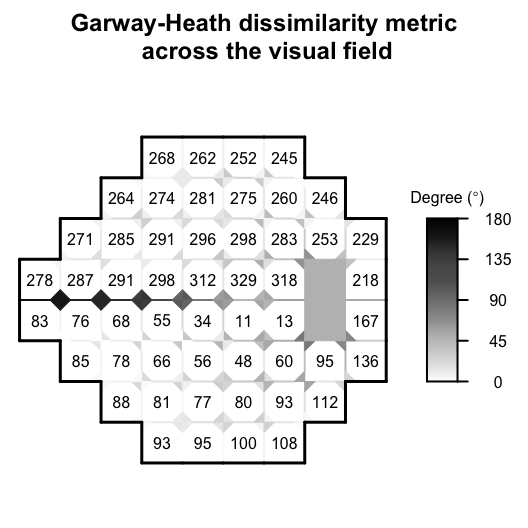Now that we have specified the data objects Y, DM, W and Time, we will customize the objects that characterize Bayesian Markov chain Monte Carlo (MCMC) methods, in particular hyperparameters, starting values, metroplis tuning values and MCMC inputs.

MCMC Characteristics

We begin be specifying the hyperparameters for the model. The parameter $$\phi$$ is uniformly distributed with bounds, $$a_{\phi}$$ and $$b_{\phi}$$. The bounds for $$\phi$$ cannot be specified arbitrarily since it is important to account for the magnitude of time elapsed. We specify the following upper and lower bounds for $$\phi$$ to dictate temporal correlation close to independence or strong correlation, resulting in a weakly informative prior distribution.

TimeDist <- abs(outer(Time, Time, "-"))
TimeDistVec <- TimeDist[lower.tri(TimeDist)]
minDiff <- min(TimeDistVec)
maxDiff <- max(TimeDistVec)
PhiUpper <- -log(0.01) / minDiff # shortest diff goes down to 1%
PhiLower <- -log(0.95) / maxDiff # longest diff goes up to 95%

Then, we can create a hyperparameters list object, Hypers, that can be used for STBDwDM.

Hypers <- list(Delta = list(MuDelta = c(3, 0, 0), OmegaDelta = diag(c(1000, 1000, 1))),
T = list(Xi = 4, Psi = diag(3)),
Phi = list(APhi = PhiLower, BPhi = PhiUpper))

Here, $$\delta$$ has a multivariate normal distribution with mean parameter $$\boldsymbol{\mu}_{\delta}$$ and covariance, $$\boldsymbol{\Omega}_{\delta}$$ and $$\mathbf{T}$$ has an inverse-Wishart distribution with degrees of freedom $$\xi$$ and scale matrix, $$\Psi$$ (See the help manual for STBDwDM for further details).

Specify a list object, Starting, that contains the starting values for the hyperparameters.

Starting <- list(Delta = c(3, 0, 0), T = diag(3), Phi = 0.5)

Provide tuning parameters for the metropolis steps in the MCMC sampler.

Nu <- length(Time) # calculate number of visits
Tuning <- list(Theta2 = rep(1, Nu), Theta3 = rep(1, Nu), Phi = 1)

We set Tuning to the default setting of all ones and let the pilot adaptation in the burn-in phase tune the acceptance rates to the appropriate range. Finally, we set the MCMC inputs using the MCMC list object.

MCMC <- list(NBurn = 10000, NSims = 10000, NThin = 10, NPilot = 20)

We specify that our model will run for a burn-in period of 10,000 scans, followed by 10,000 scans after burn-in. In the burn-in period there will be 20 iterations of pilot adaptation evenly spaced out over the period. Finally, the final number of samples to be used for inference will be thinned down to 1,000 based on the thinning number of 10. We suggest running the sampler 250,000 iterations after burn-in, but in the vignette we are limited by compilation time.

Spatiotemporal boundary dection with dissimilarity metric model

We have now specified all model objects and are prepared to implement the STBDwDM regression object. To demonstrate the STBDwDM object we will use all of its options, even those that are being used in their default settings.

reg.STBDwDM <- STBDwDM(Y = Y, DM = DM, W = W, Time = Time,
Starting = Starting, Hypers = Hypers, Tuning = Tuning, MCMC = MCMC,
Family = "tobit",
TemporalStructure = "exponential",
Distance = "circumference",
Weights = "continuous",
Rho = 0.99,
ScaleY = 10,
ScaleDM = 100,
Seed = 54)
## Burn-in progress:  |*************************************************|
## Sampler progress:  0%..  10%..  20%..  30%..  40%..  50%..  60%..  70%..  80%..  90%..  100%..

The first line of arguments are the data objects, Y, DM, W, and Time. These objects must be specified for STBDwDM to run. The second line of objects are the MCMC characteristics objects we defined previously. These objects do not need to be defined for STBDwDM to function, but are provided for the user to custimize the model to their choosing. If they are not provided, defaults are given. Next, we specify that Family be equal to tobit since we know that visual field data is censored. Furthermore, we specify TemporalStructure to be the exponential temporal correlation structure. Our distance metric on the visual field is based on the circumference of the optic disc, so we define Distance to be circumference. Then, the adjacency weights are specified to be continuous, as opposed to the binary specification of Lee and Mitchell (2011). Finally, we define the following scalar variables, Rho, ScaleY, ScaleDM, and Seed, which are defined in the manual for STBDwDM.

The following are the returned objects from STBDwDM.

names(reg.STBDwDM)
##   "mu"         "tau2"       "alpha"      "delta"      "T"
##   "phi"        "metropolis" "datobj"     "dataug"     "runtime"

The object reg.STBDwDM contains raw MCMC samples for parameters $$\mu_t$$ (mu), $$\tau_t^2$$ (tau2), $$\alpha_{tGH}$$ (alpha), $$\boldsymbol{\delta}$$ (delta), $$\mathbf{T}$$ (T) and $$\phi$$ (phi), metropolis acceptance rates and final tuning parameters (metropolis) and model runtime (runtime). The objects datobj and dataug can be ignored as they are for later use in secondary functions.

Assessing model convergence

Before analyzing the raw MCMC samples from our model we want to verify that there are no convergence issues. We begin by loading the coda package.

library(coda)

Then we convert the raw STBDwDM MCMC objects to coda package mcmc objects.

Mu <- as.mcmc(reg.STBDwDM$mu) Tau2 <- as.mcmc(reg.STBDwDM$tau2)
Alpha <- as.mcmc(reg.STBDwDM$alpha) Delta <- as.mcmc(reg.STBDwDM$delta)
T <- as.mcmc(reg.STBDwDM$T) Phi <- as.mcmc(reg.STBDwDM$phi)

We begin by checking traceplots of the parameters. For conciseness, we present one traceplot for each parameter type.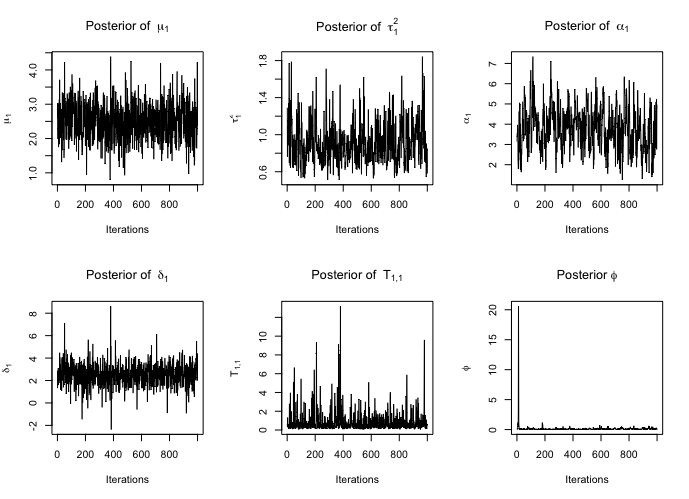From the figure, it is clear that the traceplots exhibit some poor behavior. However, these traceplots are nicely behaved considering the number of iterations the MCMC sampler ran. The traceplots demonstrate that the parameters have converged to their stationary distribution, but still need more samples to rid themselves of autocorrelation. Finally, we present the corresponding test statistics from the Geweke diagnostic test.

##       mu1     tau21    alpha1    delta1       t11       phi
## 0.8859090 0.6506118 0.4813725 0.7604529 0.8876281 0.9209661

Since none of these test statistics are terribly large in the absolute value there is not strong evidence that our model did not converge.

Post model fit analysis

Once we have verified that we do not have any convergence issues, we can begin to think about analyzing the raw MCMC samples. A nice summary for STBDwDM is to plot the posterior mean of each of the level 1 parameters over time.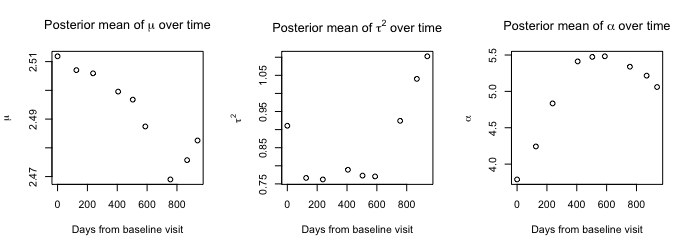This figure gives a nice summary of the model findings. In particular, the plot of the $$\alpha_{tGH}$$ demonstrate a non-linear trend and the capabilty of STBDwDM to smooth temporal effects. We now demonstrate how to calculate the posterior distribution of the coefficient of variation (cv) of $$\alpha_{tGH}$$.

CVAlpha <- apply(Alpha, 1, cv <- function(x) sd(x) / mean(x))
STCV <- c(mean(CVAlpha), sd(CVAlpha), quantile(CVAlpha, probs = c(0.025, 0.975)))
names(STCV)[1:2] <- c("Mean", "SD")
print(STCV)
##       Mean         SD       2.5%      97.5%
## 0.19087953 0.10325070 0.04504396 0.40886479

STCV (i.e., the posterior mean) was shown to be predictive of glaucome progression, so it is important to be able to compute this value. Here STCV is calculated to be 0.19.

Another component of the model that is important to explore are the adjacencies themselves, $$w_{ij}$$. As a function of $$\alpha_{tGH}$$ these adjacencies can be calculated generally, and the womblR function has provided a function PosteriorAdj to compute them.

The function PosteriorAdj function takes in the STBDwDM regression object and returns a PosteriorAdj object that contains the posterior mean and standard deviation for each adjacency at each visit.

Wij[1:6, 1:7]
##      i j DM     mean1        sd1     mean2        sd2
## [1,] 1 2  6 0.7981359 0.04875009 0.7765727 0.04479334
## [2,] 2 3 10 0.6881660 0.06995050 0.6573147 0.06274004
## [3,] 3 4  7 0.7689777 0.05477419 0.7447670 0.05001735
## [4,] 1 5  4 0.8600777 0.03505531 0.8445508 0.03262409
## [5,] 1 6  6 0.7981359 0.04875009 0.7765727 0.04479334
## [6,] 2 6 12 0.6393951 0.07794788 0.6050696 0.06911669

For visual field data, the function PlotAdjacency can be used to plot the mean and standard deviations of the adjacencies at each of the visits over the visual field surface. We plot the mean adjacencies at visit 3.

ColorScheme1 <- c("Black", "#636363", "#bdbdbd", "#f0f0f0", "White")
PlotAdjacency(Wij, Visit = 3, stat = "mean",
main = "Posterior mean adjacencies at \n visit 3 across the visual field",
color.scheme = ColorScheme1)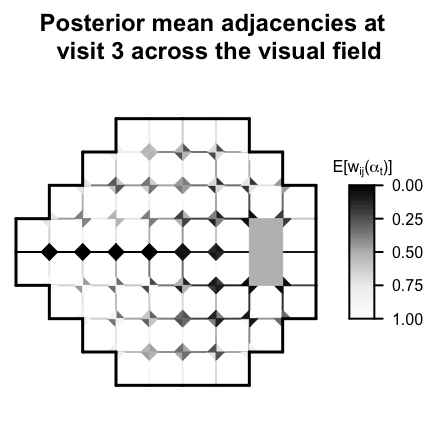And now, we plot the standard deviation of the adjacencies at visit 4.

ColorScheme2 <- rev(ColorScheme1)
zlimSD <- quantile(Wij[,c(5,7,9,11,13,15,17,19,21)], probs = c(0, 1))
PlotAdjacency(Wij, Visit = 4, stat = "sd",
main = "Posterior SD of adjacencies at \n visit 4 across the visual field",
zlim = zlimSD, color.scheme = ColorScheme2)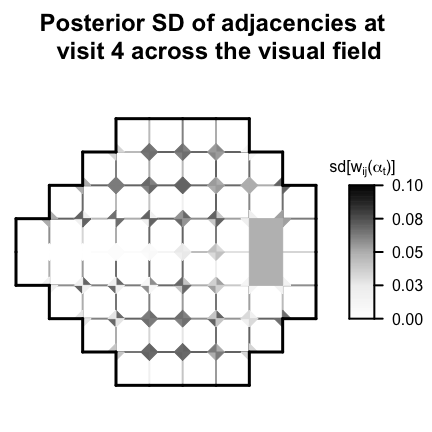The function PlotAdjacency provides a visual tool for assessing change on the visual field over time.

Compute diagnostics

The diagnostics function in the womblR package can be used to calculate various diagnostic metrics. The function takes in the STBDwDM regression object.

Diags <- diagnostics(reg.STBDwDM, diags = c("dic", "dinf", "waic"), keepDeviance = TRUE)
## Calculating Log-Lik: 0%.. 25%.. 50%.. 75%.. 100%.. Done!
## Calculating PPD: 0%.. 25%.. 50%.. 75%.. 100%.. Done!

The diagnostics function calculates diagnostics that depend on both the log-likelihood and posterior predictive distribtuion. So, if any of these diagnostics are specified, one or both of these must be sampled from. The keepDeviance and keepPPD indicate whether or not these distributions should be saved for the user. We indicate that we would like the output to be saved for the log-likelihood (i.e., deviance). We explore the output by looking at the traceplot of the deviance.

Deviance <- as.mcmc(Diags$deviance) traceplot(Deviance, ylab = "Deviance", main = "Posterior Deviance")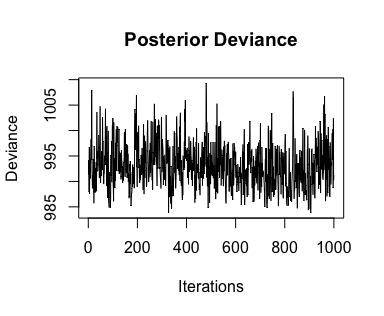This distribution has converged nicely, which is not surprising, given that the other model parameters have converged. Now we can look at the diagnostics. print(Diags) ## dic pd ## 1001.049837 8.050672 ## p g dinf ## 121068.4 250009.9 371078.4 ## waic p_waic lppd p_waic_1 ## 998.839144 4.520054 -494.899518 3.200130 Future prediction The womblR package provides the predict.STBDwDM function for sampling from the posterior predictive distribution at future time points of the observed data. This is different from the posterior predictive distribution obtained from the diagnostics function, because that distribution is for the observed time points and is automatically obtained given the posterior samples from STBDwDM. In order to obtain future samples, you first need samples from the posterior distribution of the future $$\mu_t$$, $$\tau_t^2$$, and $$\alpha_t$$ parameters. The predict.STBDwDM first samples these parameters and then samples from the future distribution of the observed outcome variable, returning both. We begin by specifying the future time points we want to predict as 50 and 100 days past the most recent visit. NewTimes <- Time[Nu] + c(50, 100) / 365 Then, we use predict.STBDwDM to calculate the future posterior predictive distribution. Predictions <- predict(reg.STBDwDM, NewTimes) ## Krigging Thetas: 0%.. 25%.. 50%.. 75%.. 100%.. Done! ## Krigging Y: 0%.. 25%.. 50%.. 75%.. 100%.. Done! We can see that predict.STBDwDM returns a list containing two lists. names(Predictions) ##  "MuTauAlpha" "Y" The object MuTauAlpha is a list containing three matrices with the posterior distributions of the future level 1 parameters. names(Predictions$MuTauAlpha)
##  "mu"    "tau2"  "alpha"
head(Predictions$MuTauAlpha$alpha)
##       alpha10  alpha11
## [1,] 6.549409 5.749063
## [2,] 5.723072 5.774788
## [3,] 3.137910 2.815721
## [4,] 4.941708 5.302214
## [5,] 8.860209 9.004541
## [6,] 3.891100 4.136476

While the object Y is a list containing however many matrices correspond to the number of new future time points (here: 2).

names(Predictions$Y) ##  "y10" "y11" You can plot a heat map representation of the posterior prediction distribution using the function PlotSensitivity. PlotSensitivity(Y = apply(Predictions$Y\$y10, 2, median),
main = "Sensitivity estimate (dB) at each \n location on visual field",
legend.lab = "DLS (dB)", legend.round = 2,
bins = 250, border = FALSE)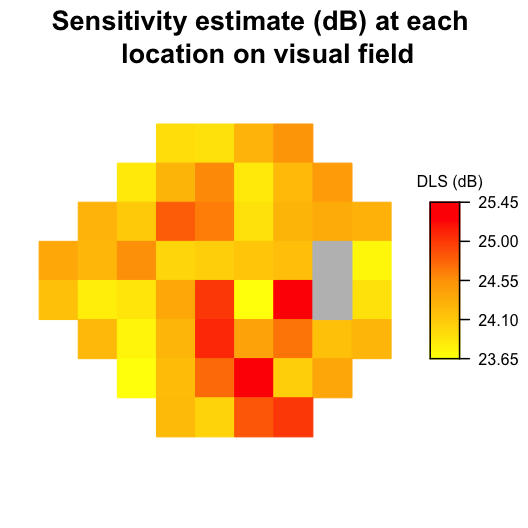This figure shows the median posterior predictive heat map over the visual field at the future visit in 50 days past the final observed visit. The PlotSensitivity function can be used for plotting any observations on the visual field surface.